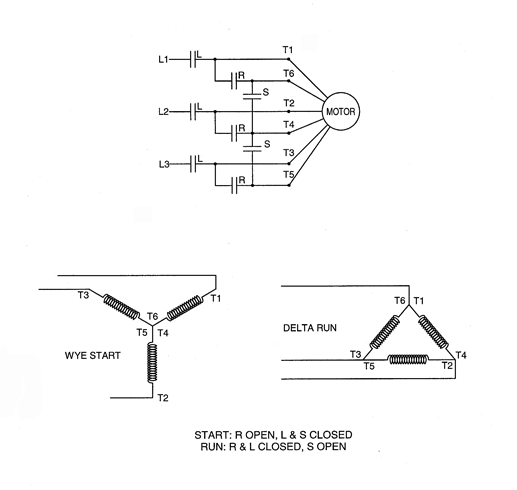WYEStart-DeltaRun
Wye Start Delta Run

This method is actually reduced voltage but is accomplished by changing the motor phase connections such that a winding that is designed to run with phase voltage equal to line voltage on delta connection is wye connected for starting to put less than line voltage on each phase. Effectively, the voltage is reduced by 1.732 factor. The impedance seen by the power system is 3 times the impedance of the delta run connection.Wye Start, Delta Run

Starting Characteristics:

1. Starting current is approximately 30% of normal.
2. Starting torque is approximately 25-30% of normal.

Applications:

1. Where load torque during acceleration is very low.
2. May be used for partial acceleration.
3. Used more often for European motors.